# GMAT Math : DSQ: Calculating arithmetic mean

## Example Questions

← Previous 1 3

### Example Question #1 : Dsq: Calculating Arithmetic Mean

Is 3 the average of a sequence？

(1)There are 3 numbers in the sequence

(2)The mode of the sequence is 3

BOTH statements TOGETHER are sufficient, but NEITHER statement ALONE is sufficient.

Statement (1) ALONE is sufficient, but statement (2) alone is not sufficient.

Statement (2) ALONE is sufficient, but statement (1) alone is not sufficient.

Statements (1) and (2) TOGETHER are NOT sufficient.

EACH statement ALONE is sufficient.

Statements (1) and (2) TOGETHER are NOT sufficient.

Explanation:

For statement (1), since we don’t know the value of each number, we cannot calculate the average of the sequence. For statement (2), the mode of the sequence is 3 means that “3” occurs most times in the sequence, but we cannot get to the average because we don’t know the value of other numbers. If we look at the two conditions together, we will know that there are 2 or 3 “3”'s in the sequence, but we don’t know exactly how many times “3” occurs. If the 3 numbers are all “3”s, then we can answer the question; If not, then we cannot answer the question. Thus both statements together are not sufficient.

### Example Question #1 : Dsq: Calculating Arithmetic Mean

Calculate the average of the 5 integers.

Statement 1: They are consecutive even integers.

Statement 2: The smallest of the integers is 8 less than the largest of the five integers.

BOTH statements TOGETHER are sufficient, but NIETHER statement ALONE is sufficient.

Statements 1 and 2 TOGETHER are NOT sufficient.

EACH statement ALONE is sufficient.

Statement 1 ALONE is sufficient, but statement 2 is not sufficient.

Statement 2 ALONE is sufficient, but statement 1 is not sufficient.

Statements 1 and 2 TOGETHER are NOT sufficient.

Explanation:

We are looking for an average here. Statement 1 tells us we are looking for even consecutive integers such as 2, 4, 6, 8, and 10. Statement 2 tells us the difference between the smallest and largest integer; however, the difference between the largest and smallest of five consecutive even (or odd) integers will ALWAYS be 8, regardless of what 5 consecutive integers we choose; therefore the two statements don't give us enough information to solve for the average.

### Example Question #23 : Descriptive Statistics

Data sufficiency question- do not actually solve the question

Find the mean of a set of 5 numbers.

1. The sum of the numbers is 72.

2. The median of the set is 15.

Each statement alone is sufficient

Statements 1 and 2 are not sufficient, and additional information is required to answer the question

Statement 2 alone is sufficient, but statement 1 is not sufficient to answer the question

Both statements taken together are sufficient to answer the question, but neither question alone is sufficient

Statement 1 alone is sufficient, but statement 2 is not sufficient to answer the question

Statement 1 alone is sufficient, but statement 2 is not sufficient to answer the question

Explanation:

Statement 2 does not provide enough information about the mean as it can vary greatly from the median. Statement 1 is sufficient to calculate the mean, because even though it is impossible to calculate the set of numbers, the mean is calculated by dividing the sum by the total number of incidences in the set.

### Example Question #3281 : Gmat Quantitative Reasoning

How much greater is the average of the integers from 500 to 700 than the average of the integers from 60 to 90?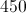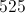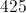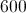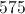Explanation:

In this case, average is also the middle value.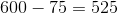### Example Question #1172 : Data Sufficiency Questions

What is the arithmetic mean of a data set with twenty data values, each of which is a positive integer?

1) The sum of the odd values is 1,144 and the sum of the even values is 856.

2) The sum of the lowest ten values is 400 and the sum of the greatest ten values is 1,600.

Statement 2 ALONE is sufficient, but Statement 1 alone is not sufficient.

EACH statement ALONE is sufficient.

Statements 1 and 2 TOGETHER are not sufficient.

Statement 1 ALONE is sufficient, but Statement 2 alone is not sufficient.

BOTH statements TOGETHER are sufficient, but neither statement ALONE is sufficient.

EACH statement ALONE is sufficient.

Explanation:

The arithmetic mean of a data set is the sum of the values divided by the number of values in the set. Since we know that there are 20 values, all we need is the sum of the values. The sum can be easily deduced to be 2,000 from either one of the statements, so the arithmetic mean can be determined to be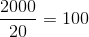.

The answer is that either statement alone is sufficient.

### Example Question #1173 : Data Sufficiency Questions

In western North Carolina, three towns lie along scenic rte 129 (the old Tallulah road). From north to south they are - Bear Creek, Sweet Gum and Robbinsville. Each town boasts of 6 establishments that sell moonshine (18 establishments total). Henry decides to test the alcohol content in all 18 establishemnts to see if there is a significant difference between the proof level in each town. He gathers the folloiwng evidence -

Robbinsville - 171, 170, 166, 180, 170, 177; avg=172.3; variance=26.67

Sweet Gum - 181, 177, 164, 190, 181, 180; avg = 178.8; variance=71.77

Bear Creek - 170, 180, 171, 191, 188, 188; avg=181.3; variance=83.87

The null hypothesis is: the 3 averages are the same.

Use the ANOVA F-test to see if we can reject the null hypothesis at the 95% level of confidence. Give both the F value and the percentile value (p) for the test.

Hint - ANOVA compares the variation between samples (MSB) to the variation within samples (MSW). If MSB is much greater than MSW, then we reject the null hypothesis and conclude that the 3 samples are significantly different. The F statistic is calculated MSB/MSW.

F=4.1

p=0.04

F=3.68

p=0.05

F=2.81

p=0.10

F=4.6

p=0.02

F=2.13

p=0.15

F=2.13

p=0.15

Explanation:

The number of sample for each town = 6; N=6; E = 6-1 = 5

Overall average (of all 18 establishments) = 177.5 = O

Number of towns (columns) = 3; V1=3-1=2

total samles = 18; V2=18-3 = 15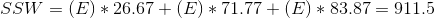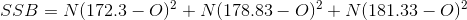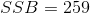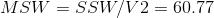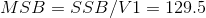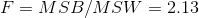For an F value of 2.13 with V1=2 and V2 = 15, p = .153

So, we can not reject the null hypothesis because our data would occur 15% of the time assuming the 3 averages are equal.

Note - the cutoff F value for 95% is 3.68

### Example Question #1174 : Data Sufficiency Questions

When assigning a score for the term, a professor takes the mean of all of a student's test scores.

Joe is trying for a score of 90 for the term. He has one test left to take. What is the minimum that Joe can score and achieve his goal?

Statement 1: He has a median score of 85 so far.

Statement 2: He has a mean score of 87 so far.

Statement 1 ALONE is sufficient to answer the question, but Statement 2 ALONE is NOT sufficient to answer the question.

BOTH statements TOGETHER are insufficient to answer the question.

EITHER statement ALONE is sufficient to answer the question.

BOTH statements TOGETHER are sufficient to answer the question, but NEITHER statement ALONE is sufficient to answer the question.

Statement 2 ALONE is sufficient to answer the question, but Statement 1 ALONE is NOT sufficient to answer the question.

BOTH statements TOGETHER are insufficient to answer the question.

Explanation:

Knowing the median score is neither necessary nor helpful. What will be needed is the sum of the scores so far and the number of tests Joe has taken. But the number of tests taken is not given, and without this, there is no way to find the sum either.

### Example Question #1175 : Data Sufficiency Questions

What is the mean of,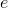, and?

Statement 1: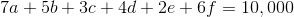Statement 2: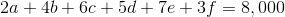Statement 2 ALONE is sufficient to answer the question, but Statement 1 ALONE is NOT sufficient to answer the question.

BOTH statements TOGETHER are insufficient to answer the question.

BOTH statements TOGETHER are sufficient to answer the question, but NEITHER statement ALONE is sufficient to answer the question.

EITHER statement ALONE is sufficient to answer the question.

Statement 1 ALONE is sufficient to answer the question, but Statement 2 ALONE is NOT sufficient to answer the question.

BOTH statements TOGETHER are sufficient to answer the question, but NEITHER statement ALONE is sufficient to answer the question.

Explanation:

The mean of a data set requires you to know the sum of the elements and the number of elements; you know the latter, but neither statement alone provides any clues to the former.

However, if you know both, you can add both sides of the equations as follows: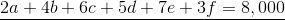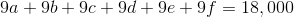Rewrite as: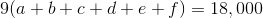and divide by 9: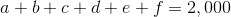Now you know the sum, so divide it by 6 to get the mean: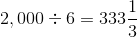.

### Example Question #1176 : Data Sufficiency Questions

A meteorologist is attempting to calculate the average (arithmetic mean)  temperature highs for the past week. What is the arithmetic mean of the high temperatures for the last week?

1. The mean high temperature for the past 3 days is 75 degrees. The mean high temperature for the 4 days before that was 80 degrees.

2. The high temperatures for the last 7 days are as follows (in degrees): 80, 81, 79, 80, 77, 75, 73

Statement (1) ALONE is sufficient, but statement (2) alone is not sufficient.

Statement (2) ALONE is sufficient, but statement (1) alone is not sufficient.

Statement (1) and (2) TOGETHER are NOT sufficient to answer the question asked, and additional data are needed.

EACH statement ALONE is sufficient.

BOTH statements TOGETHER are sufficient, but NEITHER statement ALONE is sufficient.

EACH statement ALONE is sufficient.

Explanation:

Statement 1 can be used to find the arithmetic mean by combining the information. For statement 1, the average for the last seven days is found by reversing the arithmetic mean equation. Letbe the sum of the degrees for the past 3 days. Letbe the sum of the degrees for the 4 days before that. Then we get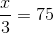and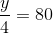so we can solve and find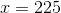and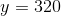.

Also, we know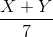= the arithmetic mean for the last week.

So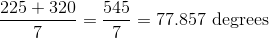Statement 2 can be used to find the arithmetic mean using the arithmetic mean formula. This is the total sum, divided by the number of days. Thus,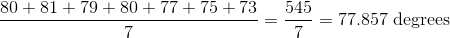### Example Question #1177 : Data Sufficiency Questions

If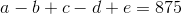and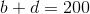, then what is the mean of, and?

It is impossible to tell from the information given.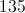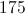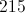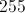Explanation:

Multiply the second equation by 2 on each side, and add it to the first equation.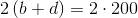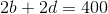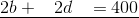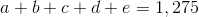Divide this sum by 5: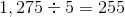← Previous 1 3

Tired of practice problems?

Try live online GMAT prep today.